# Cauchy test

The Cauchy criterion for the convergence of a series: Given a series $\sum_{n=1}^{\infty}u_n$ with non-negative real terms, if there exists a number $q$, $0\leq q<1$, such that, for all sufficiently large $n$, one has the inequality $(u_n)^{1/n}\leq q$, which is equivalent to the condition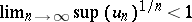, then the series is convergent. Conversely, if for all sufficiently largeone has the inequality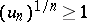, or even the weaker condition: There exists a subsequence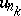,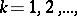with terms satisfying the inequality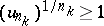, then the series is divergent.
In particular, if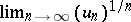exists and is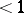, then the series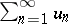is convergent; if it is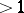, then the seriesis divergent. This was proved by A.L. Cauchy . In the case of a serieswith terms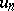of arbitrary sign, the condition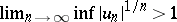implies that the series is divergent; if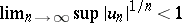, the series is absolutely convergent.
The integral Cauchy test, or the Cauchy–MacLaurin integral criterion: Given a serieswith non-negative real terms, if there exists a non-increasing non-negative function, defined for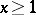, such that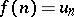,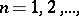then the series is convergent if and only if the integralis convergent. This test was first presented in a geometrical form by C. MacLaurin , and later rediscovered by A.L. Cauchy .